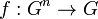# Group is n-ary group for all n

(diff) ← Older revision | Latest revision (diff) | Newer revision → (diff)
Suppose$G$ is a group where we denote the multiplication by concatenation (i.e., we omit the multiplication symbol). Let$n$ be an integer with$n \ge 2$. We can equip$G$ with the structure of a$n$-ary group (i.e., a multiary group for arity$n$) as follows: we define the$n$-ary operation$f:G^n \to G$ as:$f(a_1,a_2,\dots,a_n) = a_1a_2 \dots a_n$